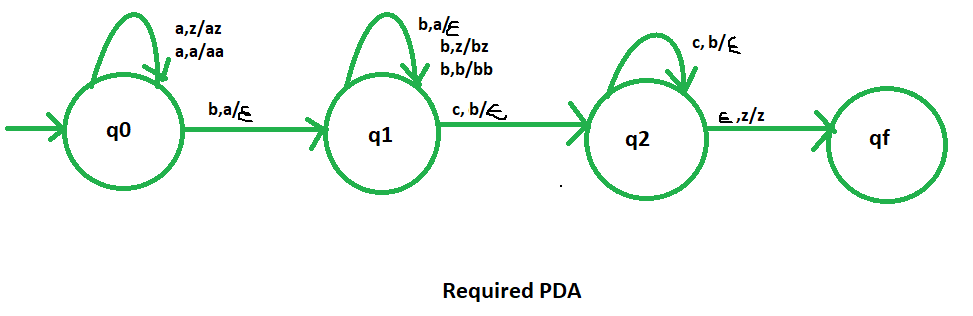# NPDA for accepting the language L = {amb(m+n)cn | m,n ≥ 1}

• Last Updated : 15 Jun, 2022

Prerequisite – Pushdown automata, Pushdown automata acceptance by final state
Problem – Design a non deterministic PDA for accepting the language L = {| m,n ≥ 1}

The strings of given language will be:

L = {abbc, abbbcc, abbbcc, aabbbbcc, ......}

In each of the string, the total sum of the number of ‘a’ and ‘c’ is equal to the number of ‘b’s. And all c’s are come after ‘a’ and ‘b’.

Explanation –
Here, we need to maintain the order of a’s, b’s and c’s. That is, all the a’s  are coming first and then all the b’s are coming after that all the c’s. Thus, we need a stack along with the state diagram. The count of a’s, b’s and c’s is maintained by the stack. We will take 3 stack alphabets:= { a, b, z }

Where,= set of all the stack alphabet
z = stack start symbol

Approach used in the construction of PDA –
As we want to design a NPDA, thus every time ‘a’ comes before ‘b’and ‘b’ comes before ‘c’.First we have to count number of a’s and that number should be equal to number of b’s. When all a’s are finished by b’s, then count number of b’s and that should be equal to number of c’s.

For all the ‘a’ we will push ‘a’ into the stack each time and then start popping them when ‘b’s are coming. After finishing all the popping of all the ‘a’s we will start pushing ‘b’ for the rest ‘b’s. And when ‘c’s are coming we will pop these ‘b’ from the stack each time. So, at the end if the stack becomes empty then we can say that the string is accepted by the PDA.

Stack transition functions –(q0, a, z)(q0, az)(q0, a, a)(q0, aa)(q0, b, a)(q1,)(q1, b, a)(q1,)(q1, b, z)(q1, bz)(q1, b, b)(q1, bb)(q1, c, b)(q2,)(q2, c, b)(q2,)(q2,, z)(qf, z)

Where, q0 = Initial state
qf = Final state= indicates pop operationMy Personal Notes arrow_drop_up
Recommended Articles
Page :